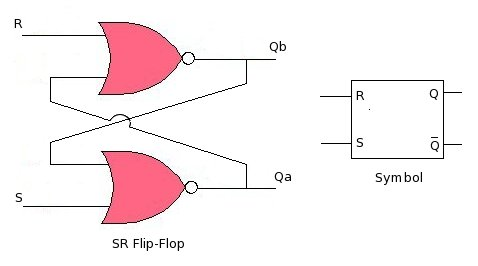black nike shoes roshe cheapnicesports.com

# Flip Flops

The Logic gates can be combined to create a two-state element. Where both the states are stable, and the element can remain in any of the state for infinite time, ideally. This two-state element is called flip-flop . A basic flip-flop and its symbol is shown in the figure below. The flip-flop shown is created with 2 NOR gates, although NAND gates can also be used. The two states being discussed are "set", and "reset" states. Voltage value at Q shows flip-flop's state, 5V means "set", and 0V means "reset". Input pins R and S are used to switch flip-flop from one state to another. Because of this property of flip-flop to latch 5V or 0V on Q, it is used as memory element, which can hold 1 bit (5V or 0V).

With circuit shown below, lets understand working of the basic flip-flop first...The circuit shown above is SR flip-flop, this is simplest one, some people also call this SR latch. Assume initially that at power-up both the inputs(R/S) are '0', Qb is 1, and Qa is 0 (both the output(Qa/Qb) can't be '0', since they are complementary). Note that Qb will be always inverted as compared to Qa, and will change simultaneously along with Qa. Now, output Qa can be set/changed by simply applying '1' (5V) at input 'S' momentarily, due to this the flipflop will change its stage to "set", Qa will become '1' and Qb will become '0' (complementary). When the flip-flop is in "set" state any input on 'S' will be ignored, and flip-flop will remain in "set" state. The only action which can switch flip-flop to "reset" state is by applying '1' (5V) momentarily at the input 'R'. When '1' is applied on 'R' momentarily, the flip-flops switches to "reset" state. In this state output Qa becomes '0', and Qb becomes '1' (complementary). When the flip-flop is in "reset" state any input on 'R' will be ignored, and flip-flop will remain in "reset" state.

Lets summaries these facts..

1. At any time flip-flop can be either in "set" or "reset" state.
2. Qa indicates state of the flip-flop, '1' on Qa means set, and '0' on Qa means "reset"
3. Flip-flop moves to "set" by momentarily setting it (as discussed above)
4. Flip-flop moves to "reset" by momentarily reseting it (as discussed above)
5. Setting a flip-flop again, which is already in "set" state won't affect the flip-flop, since in "set" state all inputs on 'S' pin are ignored.
6. Reseting a flip-flop which is already in "reset" state won't affect the flip-flop, since in "reset" state all inputs on 'R' pin are ignored
7. Please don't confuse reset or reseting discussed here with chip reset, in above discussion no where we meant chip-rest
8. Applying 1 to both 'S' and 'R' pin simultaneously will force the flip-flop to toggle rapidly between both the states, and when the inputs are removed, the final state will be unpredictable.

The table below show flip-flop's output for various inputs..

 S R Qa Qb 0 0 No change No change 0 1 0 1 1 0 1 0 1 1 Invalid Invalid

"INVALID" means for these inputs flip-flop output will be unpredictable, hence these are not allowed.

Various other flip-flops are derived from this basic concept..

1. Some have a enable pin, flip-flop changes state only if the enable pin is activated. Enable pin can be either active-low or active-high.
2. Some uses clock, where the state can be changed only when the clock is high. When clock is low, any state change activity on S or R will be ignored.
3. Some uses clock edge, here the state can be changed only when the clock edge arrives.
4. Positive edge triggered, here the state can be change only when clock's positive edge (low to high transition) arrives, all negative edges are ignored.
5. Like positive edge, negative edge flip-flops sees only -ve edges.

We will discuss some of these in more detail here.